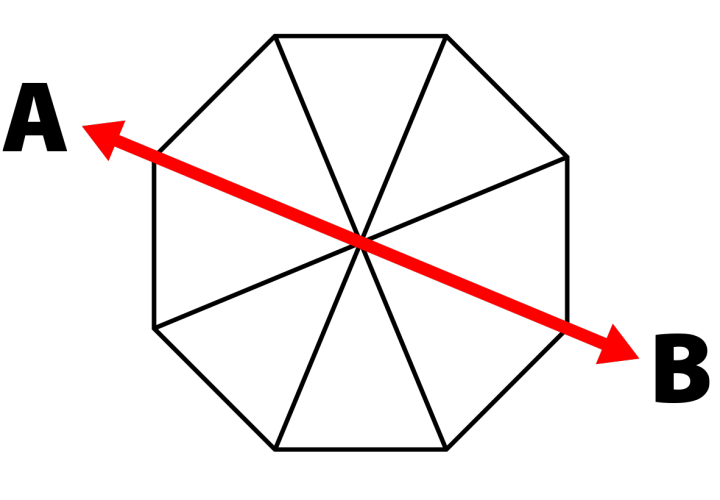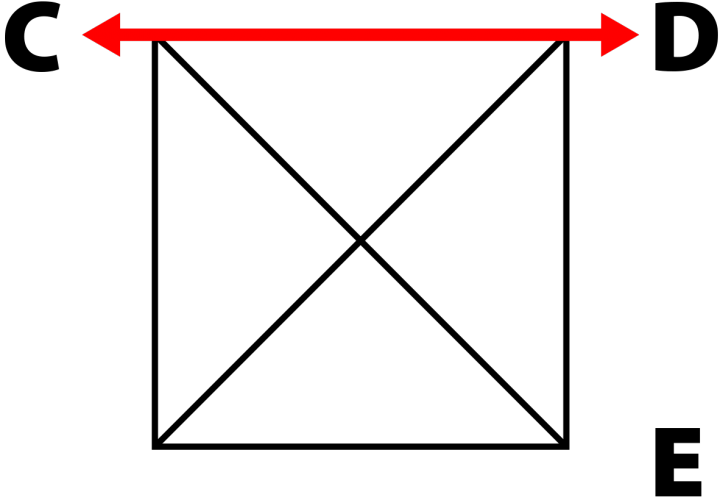# How to Measure an Umbrella

Octagon (8 Rib)

An OCTAGON (8 Rib) umbrella is measured from point A to point B diagonally along the length of the ribs.

Example: A 7.5' octagon (8 rib) umbrella would measure approximately 7.5' from point A to point B diagonally along the ribs making it a 7.5' umbrella.Square (4 Rib)

A SQUARE (4 rib) umbrella is measured from point C to point D along the edge of the panel.

Example: A 5.5' square (4 rib) would measure approximately 5.5' from point C to point D along the edge of the panel, making it a 5.5' umbrella, but measures 7.5' feet from point C to point E diagonally along the ribs.### Differences in Canopy Sizes

If you're looking for an equivlent sized umbrella in a different shape, use this to determine what you're looking for:

• 7.5' Octagon (8 Rib) Umbrella = 5.5' Square (4 Rib) Umbrella
• 9' Octagon (8 Rib) Umbrella = 6.5' Square (4 Rib) Umbrella
• 11' Octagon (8 Rib) Umbrella = 7.5' Square (4 Rib) Umbrella